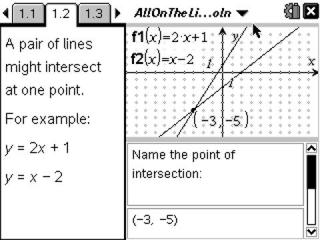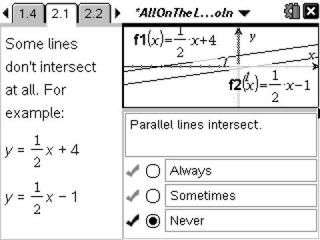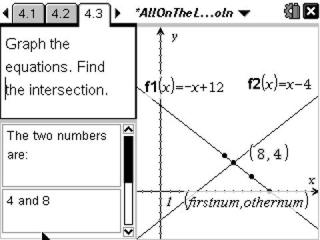# Activities

••• ##### Subject Area

• Math: Algebra I: Linear Functions

• ##### Author9-12

30 Minutes

• ##### Device
• TI-Nspire™
• TI-Nspire™ CAS
• ##### Software

TI-Nspire™
TI-Nspire™ CAS

2.0

• ##### Report an Issue

All On The Line#### Activity Overview

Students enter points into a spreadsheet and graph equations to help solve linear systems.

#### Key Steps

•In Problem 1, students will see two intersecting lines and be asked to determine the ordered pair that represents the solution. The grid is shown on the first problem, but hidden from view in the second problem to make it more challenging. Instructions are provided for students to check their answers using the Intersection Point(s) tool.

•In Problem 2, students are introduced to parallel lines in a system. Students will notice that when the slopes are equal, the lines are parallel and will not intersect. Problem 3 the case demonstrated is the same two lines with infinitely many solutions.

•For homework or an extension, students will write two equations (a system) for solving a word problem. In the spreadsheet, they will enter three pairs of numbers which are automatically displayed in a graph. Once the two linear equations are entered, the graph will reveal the solution to the problem.